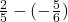## is 2/5-(-5/6) a positive, negitive, or zero

Question

is 2/5-(-5/6) a positive, negitive, or zero

in progress 0
6 months 2021-08-02T15:32:10+00:00 2 Answers 3 views 0

1. It’s a positive because if there are 2 minus or 2 negative signs it’s a negative

Positive

Step-by-step explanation:Remember, a number minus the negative of a number would be equal to that first number plus that second number but not negative. In other words:

a – (-b) = a + b

So:The sum of two positive numbers would be a positive number. Because of this, we can see that the value would be positive.

I hope you find my answer and explanation helpful. Happy studying. 🙂